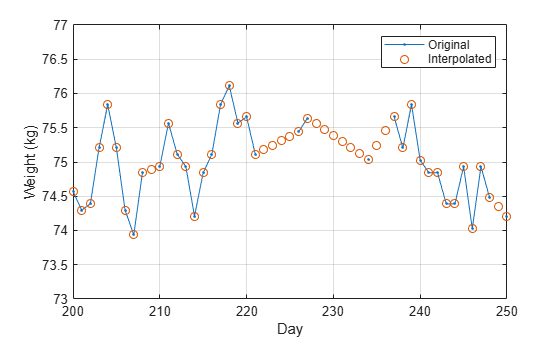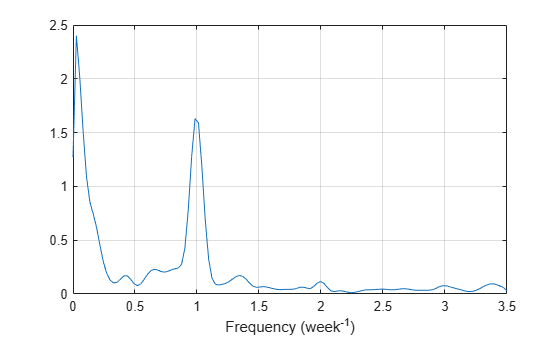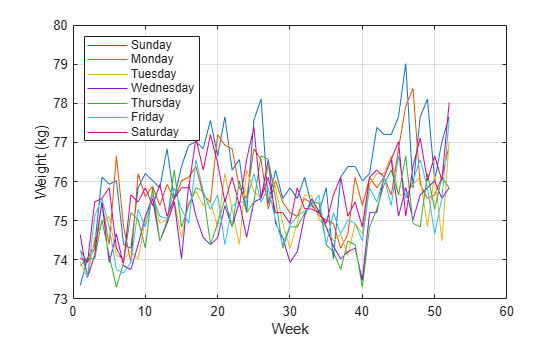Documentation

## Process a Signal with Missing Samples

Consider the weight of a person as recorded (in pounds) during the leap year 2012. The person did not record their weight every day. You would like to study the periodicity of the signal, but before you can do so you must take care of the missing data.

Load the data and convert the measurements to kilograms. Missed readings are set to `NaN`. Determine how many points are missing.

```load(fullfile(matlabroot,'examples','signal','weight2012.dat')) wgt = weight2012(:,2)/2.20462; daynum = 1:length(wgt); missing = isnan(wgt); fprintf('Missing %d samples of %d\n',sum(missing),max(daynum))```
```Missing 27 samples of 366 ```

Assign values to the missing points using `resample`. By default, `resample` makes estimates using linear interpolation. Plot the original and interpolated readings. Zoom in on days 200 through 250, which contain about half of the missing points.

```wgt_orig = wgt; wgt = resample(wgt,daynum); plot(daynum,wgt_orig,'.-',daynum,wgt,'o') xlabel('Day') ylabel('Weight (kg)') axis([200 250 73 77]) legend('Original','Interpolated') grid```Determine if the signal is periodic by analyzing it in the frequency domain. Find the cycle duration, measuring time in weeks. Subtract the mean to concentrate on fluctuations.

```Fs = 7; [p,f] = pwelch(wgt-mean(wgt),[],[],[],Fs); plot(f,p) xlabel('Frequency (week^{-1})') grid```Notice how the person's weight oscillates weekly. Is there a noticeable pattern from week to week? Eliminate the last two days of the year to get 52 weeks. Reorder the measurements according to the day of the week.

```wgd = reshape(wgt(1:7*52),[7 52]); plot(wgd') xlabel('Week') ylabel('Weight (kg)') q = legend(datestr(datenum(2012,1,1:7),'dddd')); q.Location = 'NorthWest'; grid```Smooth out the fluctuations using a filter that fits low-order polynomials to subsets of the data. Specifically, set it to fit cubic polynomials to sets of seven days.

```wgs = sgolayfilt(wgd',3,7); plot(wgs) xlabel('Week') ylabel('Weight (kg)') title('Smoothed Weight Fluctuations') q = legend(datestr(datenum(2012,1,1:7),'dddd')); q.Location = 'SouthEast'; grid```This person tends to eat more, and thus weigh more, during the weekend. Verify by computing the daily means.

```for jk = 1:7 fprintf('%3s mean: %5.1f kg\n', ... datestr(datenum(2012,1,jk),'ddd')',mean(wgd(jk,:))) end```
```Sun mean: 76.2 kg Mon mean: 75.7 kg Tue mean: 75.2 kg Wed mean: 74.9 kg Thu mean: 75.1 kg Fri mean: 75.3 kg Sat mean: 75.8 kg ```